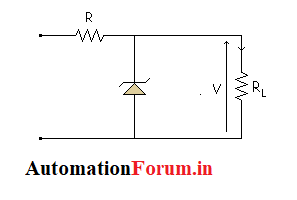# Zener diode regulator### What is zener diode regulator?

Zener diode regulator is an electronic device designed to protect electrical and electronic devices sensitive to variations in potential difference or voltage and noise in the alternating current of the electrical distribution

The zener regulator can regulate powers ranging from less than 2 volts to several hundred volts, and the power that can dissipate goes from 0.25 watts to 50 watts or more.

### Characteristics of Zener diode regulator

The power that dissipates a Zener diode is simply the multiplication of the voltage for which it was manufactured by the current flowing through it, ie: Pz = Vz x Iz

This means that the maximum current that can pass through a Zener diode is: Iz = Pz / Vz.

Iz = Current passing through the Zener diode
Pz = Power of the zener diode (manufacturer’s data) Vz = Voltage of the zener diode (manufacturer’s data)

#### Calculation of limiting resistor Rs:

The calculation of the resistor Rs is determined by the current that will be consumed by the load (the one that is connected to this voltage source).

The voltage regulator with Zener diode, with the resistor Rs connected between Vin and the cathode of the Zener

This resistor can be calculated with the following formula:

Rs = [Vmin – Vz] /1.1 x ILmax

V(min): it is the minimum value of the input voltage. (remember that it is an unregulated voltage and can vary its parameters)

IL (max): is the value of the maximum current that the load will request.

Once known Rs, the maximum power of the Zener diode is obtained, with the help of the formula:

PD = [[ Vmin-Vz ] / Rs-ILmin] x Vz

### Zener with changing input:

Zener diode regulators can provide a reasonably constant level of DC output, but they are not particularly efficient. For this reason, they are limited to applications that require only little current for the load.

As the input voltage varies (within limits), the zener diode maintains an almost constant output voltage at its terminals.

However, as the VIN changes, IZ will change proportionally, so that the limitations in the variation of the input voltage are established by the minimum and maximum current values (IZK and IZM) with which the zener can operate. The resistance R is the current limiting resistor in series. The meters indicate the relative values and trends.

Scroll to Top